## Operations with Algebraic Fractions

Many techniques will simplify your work as you perform operations with algebraic fractions. As you review the examples, note the steps involved in each operation and any methods that will save you time.

#### Reducing algebraic fractions

To reduce an algebraic fraction to lowest terms, first factor the numerator and the denominator; then reduce, (or divide out) common factors.

##### Example 1

Reduce.

1.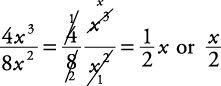2.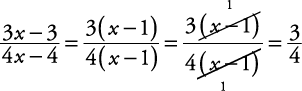3.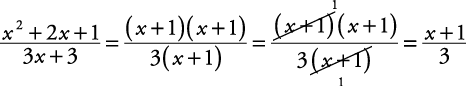Warning: Do not reduce through an addition or subtraction sign as shown here.#### Multiplying algebraic fractions

To multiply algebraic fractions, first factor the numerators and denominators that are polynomials; then, reduce where possible. Multiply the remaining numerators together and denominators together. (If you've reduced properly, your answer will be in reduced form.)

##### Example 2

Multiply.

1.2.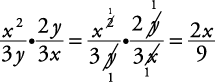3.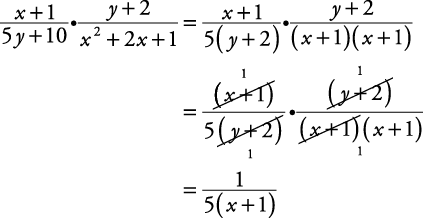4.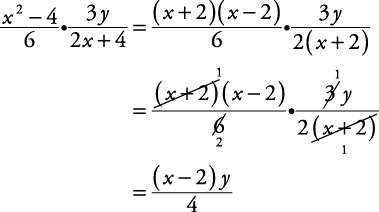5.#### Dividing algebraic fractions

To divide algebraic fractions, invert the second fraction and multiply. Remember, you can reduce only after you invert.

##### Example 3

Divide.

1.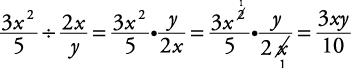2.#### Adding or subtracting algebraic fractions

To add or subtract algebraic fractions having a common denominator, simply keep the denominator and combine (add or subtract) the numerators. Reduce if possible.

##### Example 4

Perform the indicated operation.

1.2.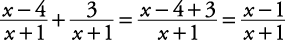3.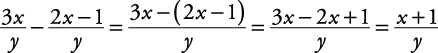To add or subtract algebraic fractions having different denominators, first find a lowest common denominator (LCD), change each fraction to an equivalent fraction with the common denominator, and then combine each numerator. Reduce if possible.

##### Example 5

Perform the indicated operation.

1.2.If there is a common variable factor with more than one exponent, use its greatest exponent.

##### Example 6

Perform the indicated operation.

1.2.3.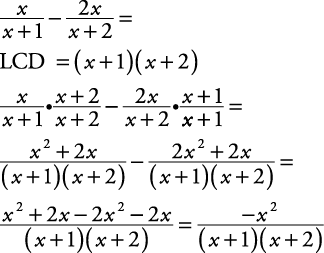To find the lowest common denominator, it is often necessary to factor the denominators and proceed as follows.

##### Example 7

Perform the indicated operation.Occasionally, a problem will require reducing what appears to be the final result. A problem like that is found in the next example.

##### Example 8

Perform the indicated operation.